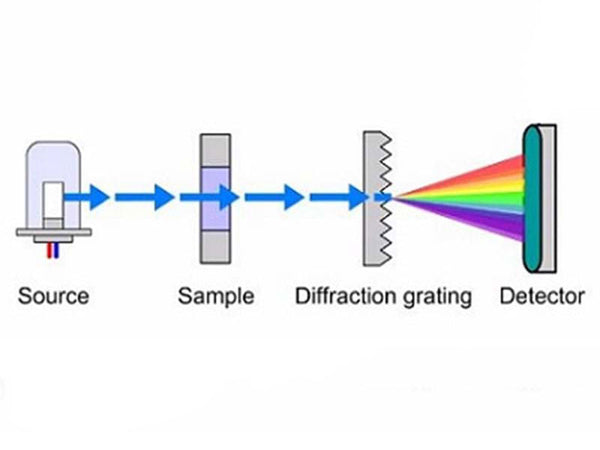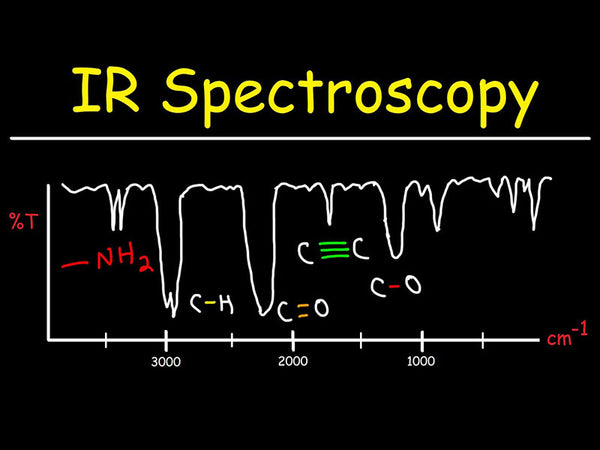Infrared spectroscopy is an indispensable method for studying the molecular structure of compounds. When a beam of infrared rays is irradiated to the sample under test, the molecule transitions from the low energy level of vibration to the adjacent high energy level, and an absorption peak appears in the region corresponding to the characteristic frequency of the group. The qualitative and quantitative data of the molecule can be obtained from the position and intensity of the absorption peak.

According to the viewpoint of quantum theory, when a substance is irradiated by incident light, when a molecule absorbs energy, the increase in its energy is jumping, so the substance can only absorb a certain energy of light quantum. The energy difference between the two energy levels and the frequency of the absorbed light obey the Bohr formula:

hv＝E2－E1

In the formula, E1 and E2 are the energy of low-energy state and high-energy state respectively; h is Planck's constant, 6.626×10-34J·s; v is the frequency of light wave.

It can be seen from the above formula that if the energy difference between the low-energy state and the high-energy state is greater, the frequency of the absorbed light is higher; on the contrary, the frequency of the absorbed light is lower. Usually frequency v can be expressed by wave number.

That quantum theory believes that a transition between two energy levels can only occur when a certain rule is followed, that is, a transition between two energy levels can only occur when the electric dipole change is equal to zero. The molecular motion can be divided into translation, rotation, vibration and the motion of electrons in the molecule, and each state of motion belongs to a certain energy level.

When the substance absorbs the light quantum in the infrared region, it can only cause the vibration of atoms and the rotation of molecules, and will not cause the beating of electrons, so infrared spectrum is also called vibration rotation spectrum.

The absorption peaks in the infrared spectrum correspond to the different vibration patterns of the molecules and the groups in the molecules. The vibration forms of molecular dipole changes can be divided into two categories: stretching vibration and bending vibration. Stretching vibration refers to the movement of atoms back and forth along the direction of the valence bond; bending vibration refers to the movement of the atom perpendicular to the direction of the valence bond.

Each atom in a molecule has 3 degrees of freedom in space, and a molecule composed of N atoms should have 3N degrees of freedom in space. For non-linear molecules, 3 translational degrees of freedom and 3 rotational degrees of freedom should be subtracted. In addition to vibrating relative to each other, all atomic nuclei can also vibrate relative to the entire molecule, so there are many vibration frequency groups. Certain vibration frequencies are related to the existence of certain groups in the molecule. The energy of the bond is different, the vibration energy absorbed is also different. Therefore, each group and chemical bond has a special absorption frequency group, which can be analyzed by infrared absorption spectroscopy, but the characteristic frequency of the same group in different molecules will slightly change, so it is necessary to pay attention to the influence of various factors when applying.

In the process of molecular vibration, the vibration frequency of the same type of chemical bond is very close. They always appear within a certain range, but are different from each other, the so-called characteristic frequency or group frequency.

The atoms in the molecule are centered at the equilibrium point and vibrate periodically with a very small amplitude (compared to the distance between the nuclei), which is the so-called simple harmonic vibration. According to this molecular vibration model, the two atoms connected by chemical bonds are approximately regarded as harmonic oscillators, then the vibration frequency of each harmonic oscillator (chemical bond) in the molecule (basic vibration frequency) can be calculated by the numbered spectrum vibration formula (also called vibration equation) derived from Hooke's law in classical mechanics:In the formula, the vibration frequency v is expressed by the wave number σ, then:In the formula, c is the speed of light; K is the chemical bond force constant (ie, chemical bond strength); μ is the reduced mass of 2 atoms, that is, μ=m1m2/(m1+m2).

According to the relationship between atomic mass and atomic weight, the above formula can be rewritten as:In the formula, N is Avogadro's constant, 6.024×1023; M is the converted atomic weight of 2 atoms, that is, M=M1M2/(M1+M2). When the unit of the chemical bond force constant K is represented by 10-2N/m, the above formula is simplified to:The above formula is the molecular vibration equation, which can be used to calculate the vibration frequency of chemical bonds in diatomic molecules or complex molecules.

The measured bond force constants of single bond, double bond and triple bond are Ksingle=(4~6)×10-5N/m, Kdouble=(8~12)×10-5N/m, Ktriple=(12~18)×10-5N/m. In this way, the vibration frequency of the corresponding chemical bond can be obtained from the known chemical bond strength.The intensity of the absorption peak of the molecular absorption spectrum can be expressed by the molar absorption coefficient ε. Since the intensity of the infrared absorption band is much weaker than that of ultraviolet and visible light, even the absorption band generated by the vibration of a strong polar group is 2 to 3 orders of magnitude lower than that of ultraviolet and visible light; and the intensity of the infrared radiation source is also weak. The intensity of the infrared absorption peak is usually roughly expressed in the following 5 levels:

VS - Very strong peak - ε＞100

S - Strong peak - ε=20~100

m - Medium strong peak - ε=10~20

W - Weak peak - ε=1~10

VW - Very weak peak - ε＜1

The peak intensity is related to the probability of molecular transition. The transition probability refers to the percentage of the total number of molecules in the excited state. The transition probability of the fundamental frequency peak is large, the transition probability of the double frequency peak is small, and the transition probability of the group frequency peak is even smaller. The peak intensity is related to the dipole moment of the molecule, and the dipole moment of the molecule is related to the polarity, symmetry of the molecule and the vibration mode of the group. Generally, molecules or groups with stronger polarity have stronger absorption peaks. The lower the symmetry of the molecule, the stronger the absorption peak; when the vibration mode of the group is different, the charge distribution is different, and the intensity of the absorption peak is generally: vas>vs>δ. Infrared spectroscopy usually takes the wavelength or wave number of the absorption band as the abscissa, and the transmittance percentage is the ordinate to express the absorption intensity.